# Electric field intensity Electric potential Membrane potential Electric

• Slides: 31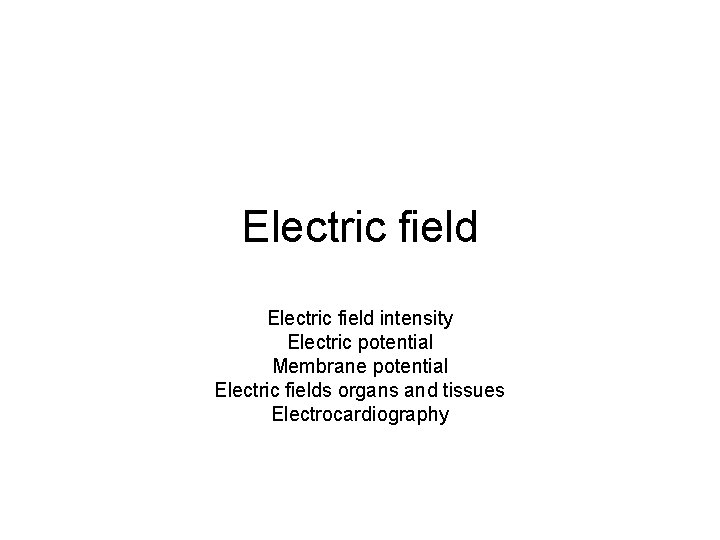Electric field intensity Electric potential Membrane potential Electric fields organs and tissues ElectrocardiographyCoulomb's Law • The electric force acting on a point charge q 1 as a result of the presence of a second point charge q 2 is given by where r - distance between charges ε 0 - permittivity of space Note that this satisfies Newton's third law because it implies that exactly the same magnitude of force acts on q 2. Coulomb's law is a vector law and includes the fact that the force acts along the line joining the charges.Electric field • Intensity of an electric field E (or strength of an electric field or electric field) is vector characteristic. It is defined as the ratio of the force F acting upon the test charge to the magnitude of the test charge: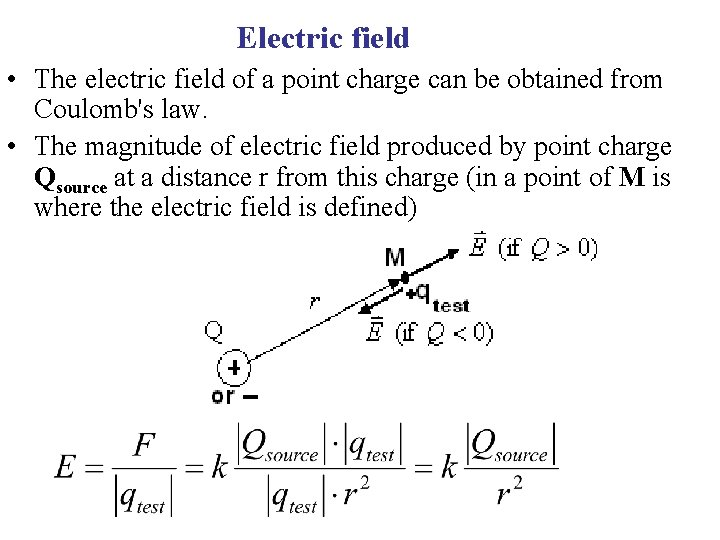Electric field • The electric field of a point charge can be obtained from Coulomb's law. • The magnitude of electric field produced by point charge Qsource at a distance r from this charge (in a point of M is where the electric field is defined)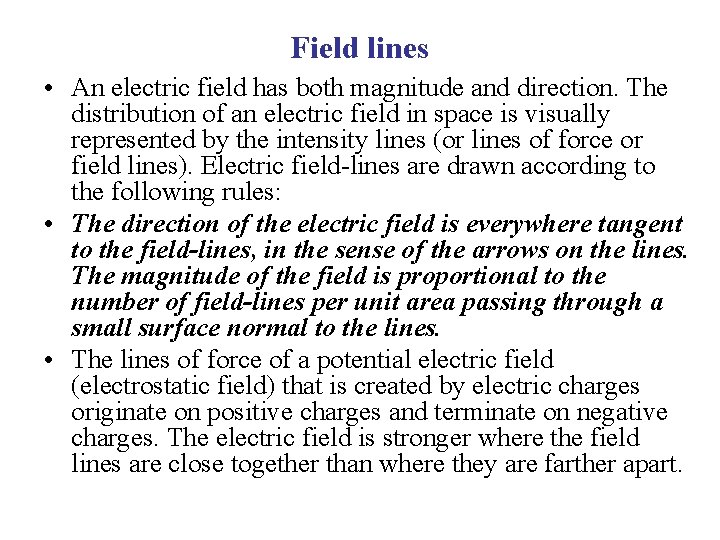Field lines • An electric field has both magnitude and direction. The distribution of an electric field in space is visually represented by the intensity lines (or lines of force or field lines). Electric field-lines are drawn according to the following rules: • The direction of the electric field is everywhere tangent to the field-lines, in the sense of the arrows on the lines. The magnitude of the field is proportional to the number of field-lines per unit area passing through a small surface normal to the lines. • The lines of force of a potential electric field (electrostatic field) that is created by electric charges originate on positive charges and terminate on negative charges. The electric field is stronger where the field lines are close together than where they are farther apart.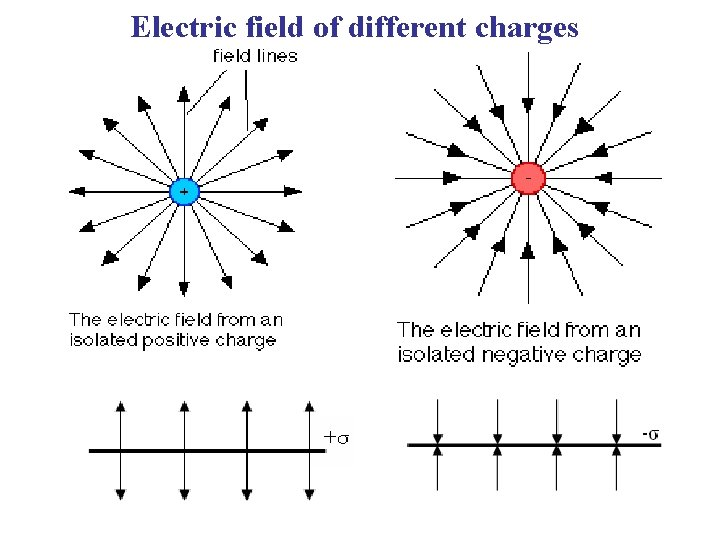Electric field of different charges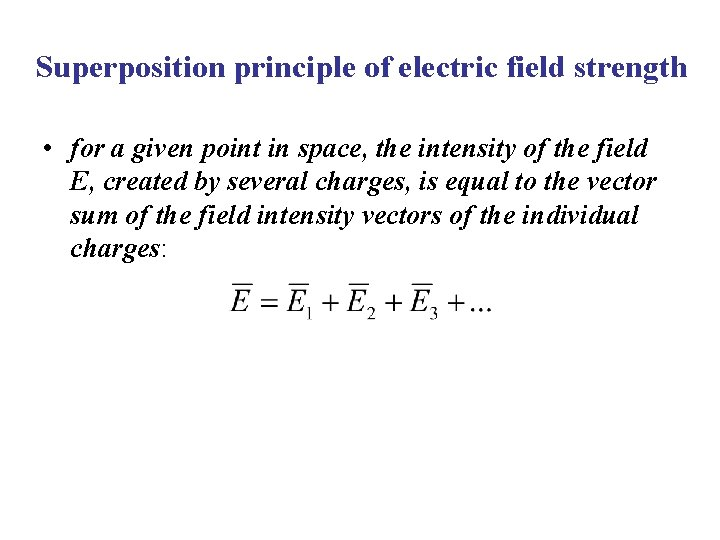Superposition principle of electric field strength • for a given point in space, the intensity of the field E, created by several charges, is equal to the vector sum of the field intensity vectors of the individual charges:Electric field between two plates charged by the same density of σ but opposite sign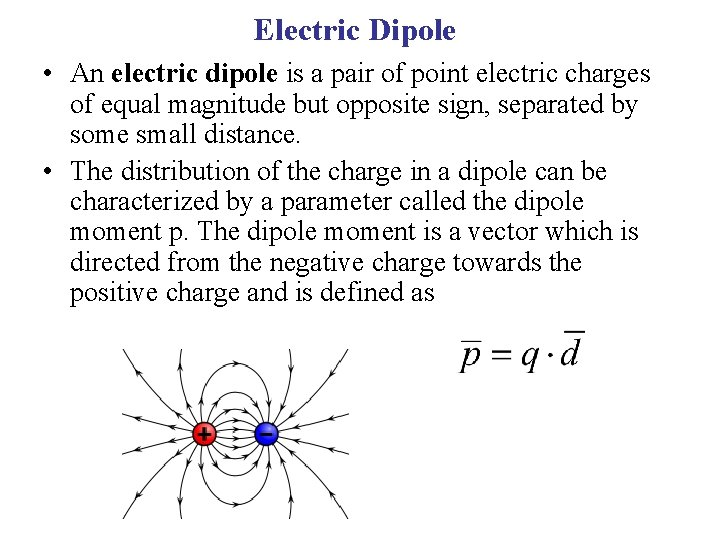Electric Dipole • An electric dipole is a pair of point electric charges of equal magnitude but opposite sign, separated by some small distance. • The distribution of the charge in a dipole can be characterized by a parameter called the dipole moment p. The dipole moment is a vector which is directed from the negative charge towards the positive charge and is defined as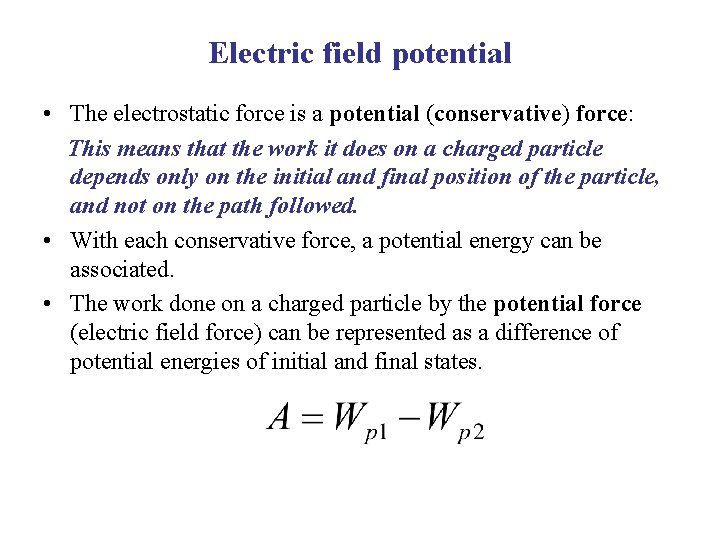Electric field potential • The electrostatic force is a potential (conservative) force: This means that the work it does on a charged particle depends only on the initial and final position of the particle, and not on the path followed. • With each conservative force, a potential energy can be associated. • The work done on a charged particle by the potential force (electric field force) can be represented as a difference of potential energies of initial and final states.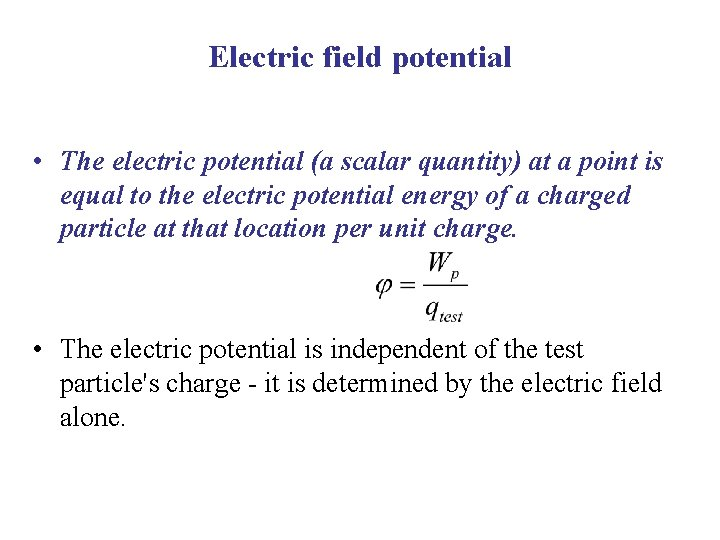Electric field potential • The electric potential (a scalar quantity) at a point is equal to the electric potential energy of a charged particle at that location per unit charge. • The electric potential is independent of the test particle's charge - it is determined by the electric field alone.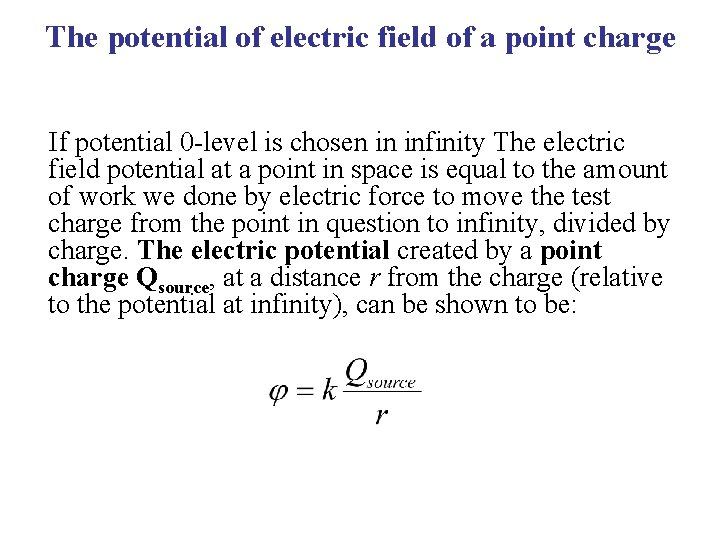The potential of electric field of a point charge If potential 0 -level is chosen in infinity The electric field potential at a point in space is equal to the amount of work we done by electric force to move the test charge from the point in question to infinity, divided by charge. The electric potential created by a point charge Qsource, at a distance r from the charge (relative to the potential at infinity), can be shown to be: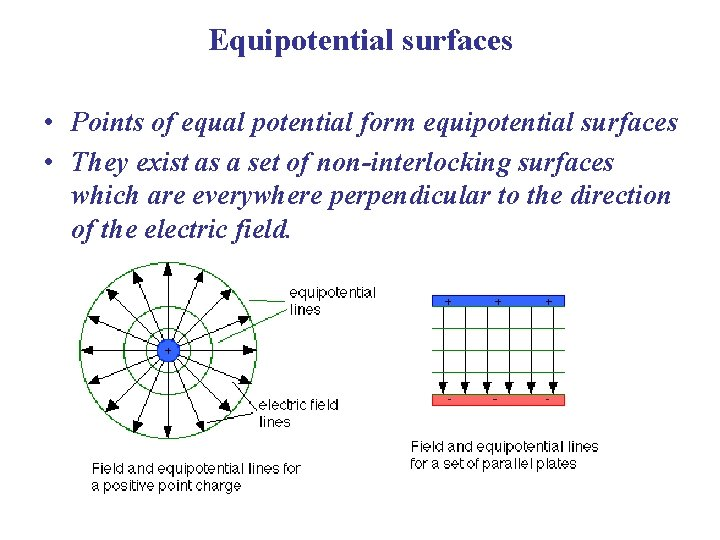Equipotential surfaces • Points of equal potential form equipotential surfaces • They exist as a set of non-interlocking surfaces which are everywhere perpendicular to the direction of the electric field.Superposition principle of electric field potential • for a given point in space, the total electric potential, created by several charges, is equal to the scalar sum of the potentials of the individual charges: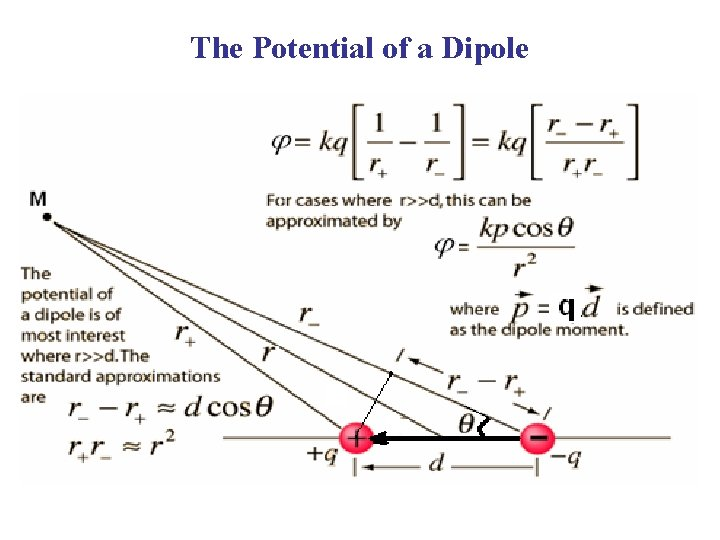The Potential of a Dipole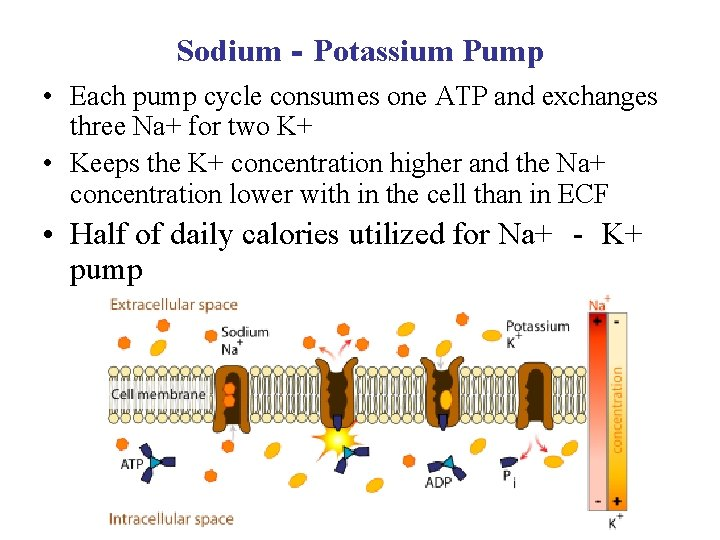Sodium‐Potassium Pump • Each pump cycle consumes one ATP and exchanges three Na+ for two K+ • Keeps the K+ concentration higher and the Na+ concentration lower with in the cell than in ECF • Half of daily calories utilized for Na+ ‐ K+ pump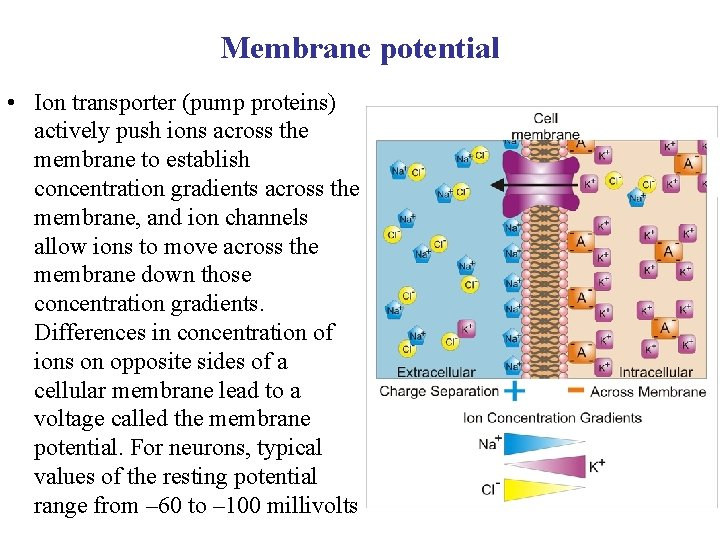Membrane potential • Ion transporter (pump proteins) actively push ions across the membrane to establish concentration gradients across the membrane, and ion channels allow ions to move across the membrane down those concentration gradients. Differences in concentration of ions on opposite sides of a cellular membrane lead to a voltage called the membrane potential. For neurons, typical values of the resting potential range from – 60 to – 100 millivoltsNernst equation for equilibrium potential for K+ ions. • Goldman–Hodgkin–Katz voltage equation (more commonly known as the Goldman equation)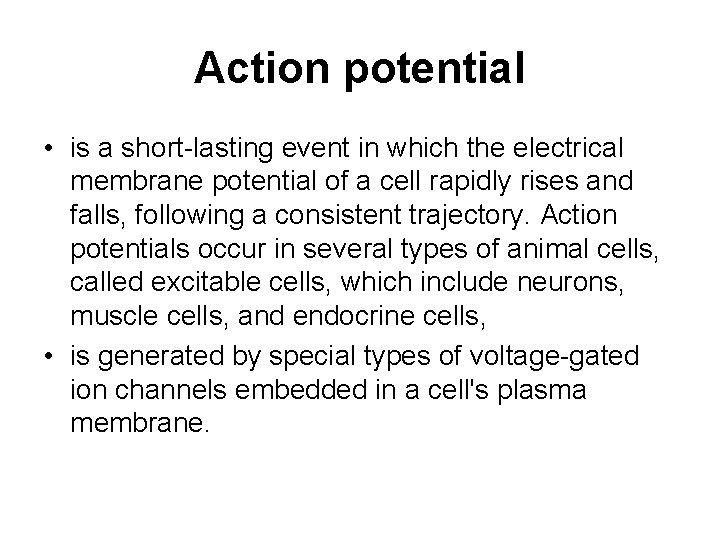Action potential • is a short-lasting event in which the electrical membrane potential of a cell rapidly rises and falls, following a consistent trajectory. Action potentials occur in several types of animal cells, called excitable cells, which include neurons, muscle cells, and endocrine cells, • is generated by special types of voltage-gated ion channels embedded in a cell's plasma membrane.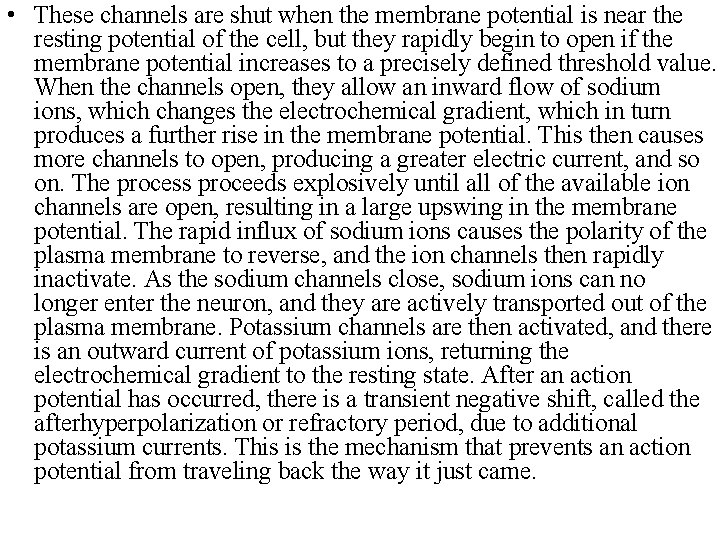• These channels are shut when the membrane potential is near the resting potential of the cell, but they rapidly begin to open if the membrane potential increases to a precisely defined threshold value. When the channels open, they allow an inward flow of sodium ions, which changes the electrochemical gradient, which in turn produces a further rise in the membrane potential. This then causes more channels to open, producing a greater electric current, and so on. The process proceeds explosively until all of the available ion channels are open, resulting in a large upswing in the membrane potential. The rapid influx of sodium ions causes the polarity of the plasma membrane to reverse, and the ion channels then rapidly inactivate. As the sodium channels close, sodium ions can no longer enter the neuron, and they are actively transported out of the plasma membrane. Potassium channels are then activated, and there is an outward current of potassium ions, returning the electrochemical gradient to the resting state. After an action potential has occurred, there is a transient negative shift, called the afterhyperpolarization or refractory period, due to additional potassium currents. This is the mechanism that prevents an action potential from traveling back the way it just came.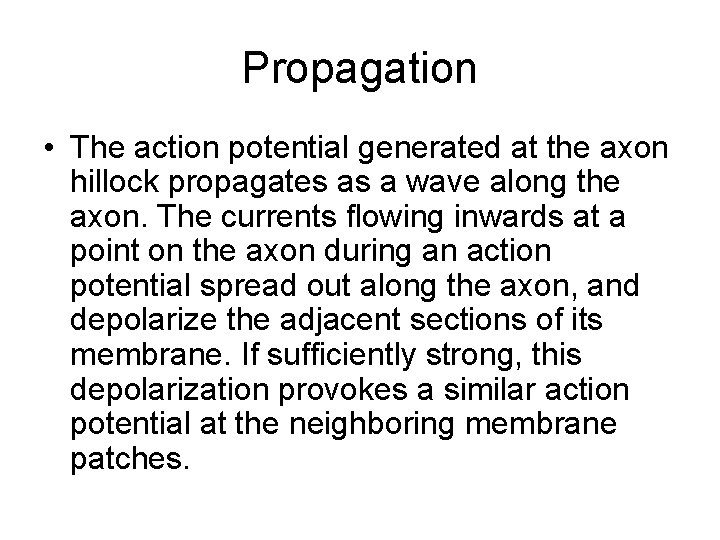Propagation • The action potential generated at the axon hillock propagates as a wave along the axon. The currents flowing inwards at a point on the axon during an action potential spread out along the axon, and depolarize the adjacent sections of its membrane. If sufficiently strong, this depolarization provokes a similar action potential at the neighboring membrane patches.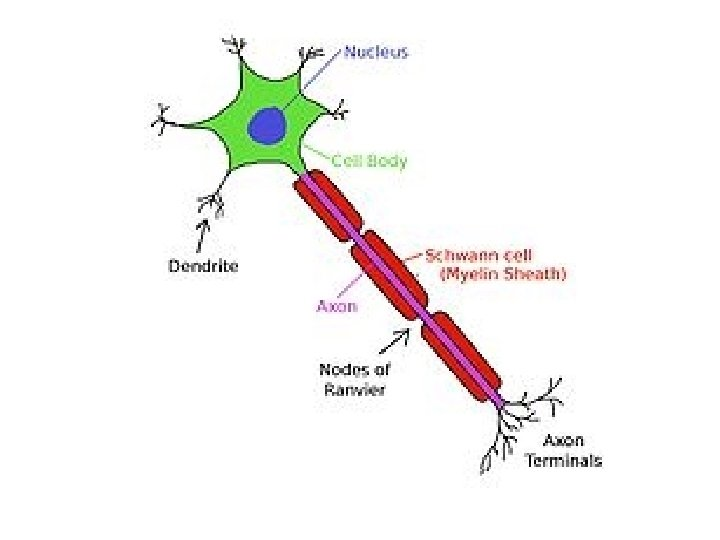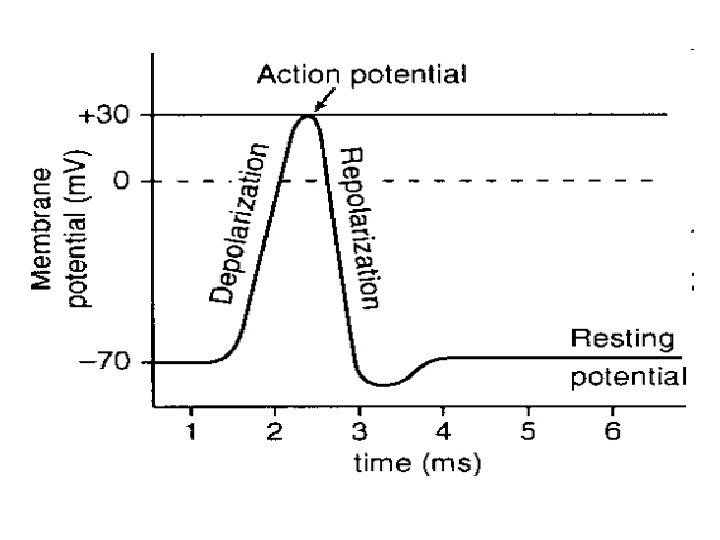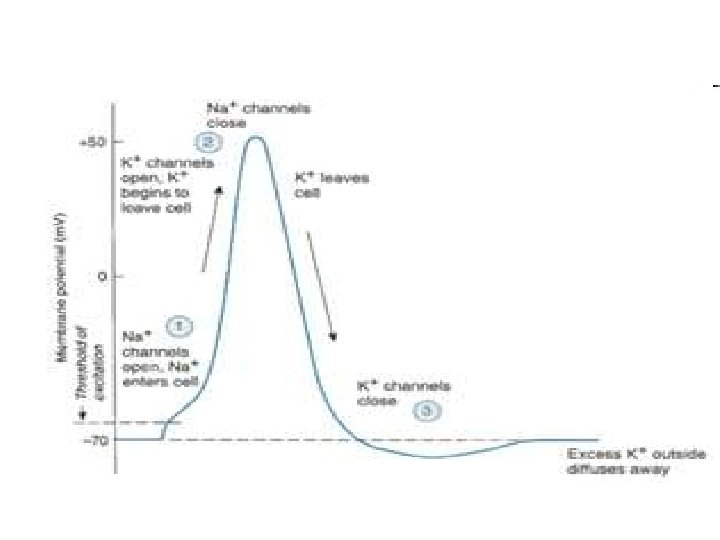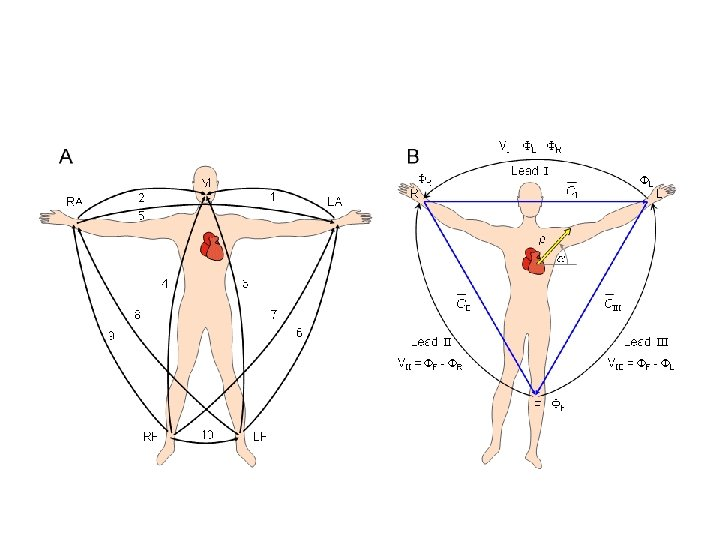Einthoven's triangle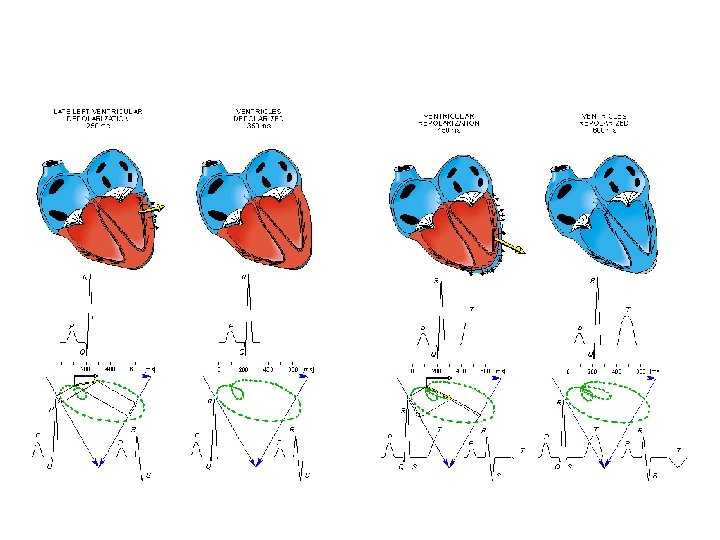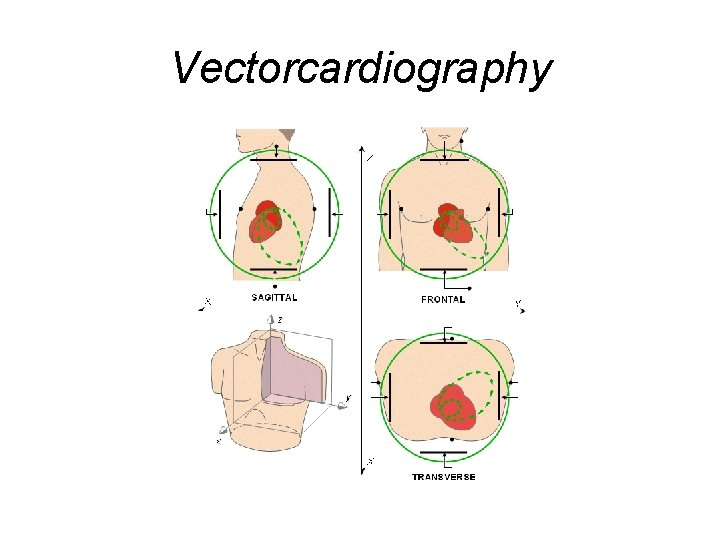Vectorcardiography